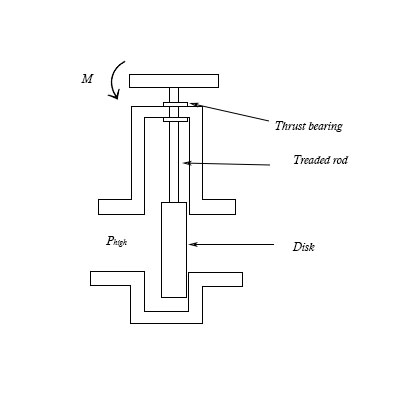# It is shown gate valve. The valve operates by applying a moment to the handle that raises a disk....

## Question:

It is shown gate valve. The valve operates by applying a moment to the handle that raises a disk. The disk has a diameter of {eq}2 in {/eq}. {eq}P_{high}= 30 psig. {/eq} The threaded rod has a diameter of {eq}0.5 in {/eq} and {eq}4 {/eq} threads per inch. The thrust bearing has an inner radius of {eq}0.5 in {/eq}. and an outer radius of {eq}1 in {/eq}. All surfaces have a coefficient of friction {eq}\mu_s=0.2 {/eq} and {eq}\mu_k=0.19. {/eq}

a. Find the force required to lift the gate valve (disk).

b. Find the moment required to overcome the friction in the threads.

c.Find the moment required to overcome the friction in the thrust bearing.## Moment

The turning effect produced by a force subjected to a body from a certain distance is called the moment of the applied force. Moment of a force is a vector quantity as it has sense of direction along with the magnitude.

## Answer and Explanation:

Given data:

• The diameter of the disk is: {eq}d = 2\;{\rm{in}} {/eq}
• The pressure is: {eq}{P_{high}} = 30\;{\rm{psig}} {/eq}
• The diameter of the threaded rod is: {eq}{d_r} = 0.5\;{\rm{in}} {/eq}
• The number of threads per inch is: {eq}n = 4 {/eq}
• The inner radius of the thrust bearing is: {eq}{r_i} = 0.5\;{\rm{in}} {/eq}
• The outer radius of the thrust bearing is: {eq}{r_o} = 1\;{\rm{in}} {/eq}
• The coefficient of static friction is: {eq}{\mu _s} = 0.2 {/eq}
• The coefficient of kinetic friction is: {eq}{\mu _k} = 0.19 {/eq}

(a)

Write the expression for the force required to lift the disk.

{eq}F = {\mu _s}{P_{high}} \times A = {\mu _s}{P_{high}} \times \frac{\pi }{4}{d^2} {/eq}

Here, the area of the disk is {eq}A {/eq}.

Substitute the values in the above equation.

{eq}\begin{align*} F &= 0.2 \times 30\;{\rm{psig}} \times \frac{\pi }{4}{\left( {2\;{\rm{in}}} \right)^2}\\ &= 18.84\;{\rm{lb}} \end{align*} {/eq}

Thus the force required is {eq}18.84\;{\rm{lb}} {/eq}.

(b)

Write the expression for the moment required to overcome friction in threads.

{eq}m = F{\mu _k} \times {d_r} {/eq}

Substitute the values in the above equation.

{eq}\begin{align*} m &= 18.84\;{\rm{lb}} \times 0.19 \times 0.5\;{\rm{in}}\\ &= 1.78\;{\rm{lb}} \cdot {\rm{in}} \end{align*} {/eq}

Thus the moment required is {eq}1.78\;{\rm{lb}} \cdot {\rm{in}} {/eq}.

(c)

Write the expression for the moment required to overcome friction in thrust bearing.

{eq}m' = F \times {\mu _s} \times \left( {\dfrac{{{d_o} + {d_i}}}{2}} \right) {/eq}

Substitute the values in the above equation.

{eq}\begin{align*} m' &= 18.84\;{\rm{lb}} \times 0.2 \times \left( {\dfrac{{1\;{\rm{in}} + 0.5\;{\rm{in}}}}{2}} \right)\\ &= 2.82\;{\rm{lb}} \cdot {\rm{in}} \end{align*} {/eq}

Thus the moment required is {eq}2.82\;{\rm{lb}} \cdot {\rm{in}} {/eq}.

#### Learn more about this topic:Torque in Physics: Equation, Examples & Problems

from Physics: Middle School

Chapter 3 / Lesson 13
43K